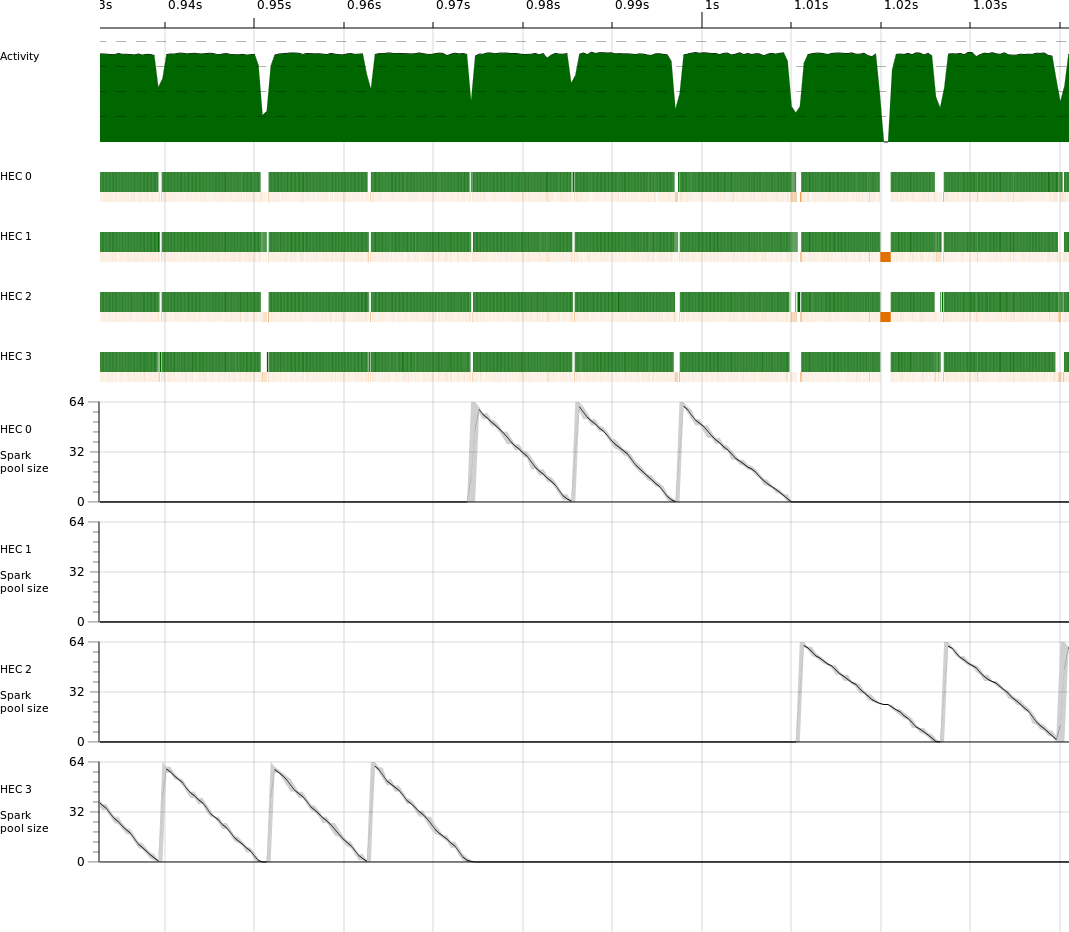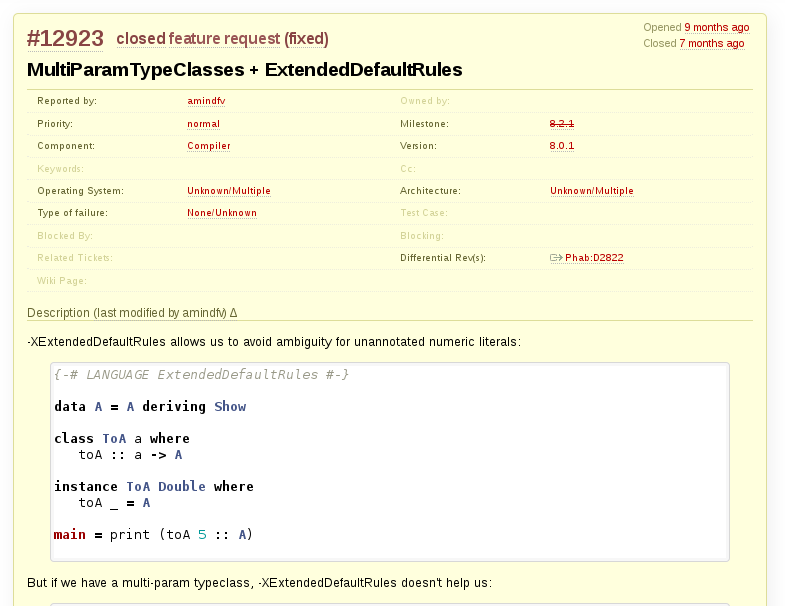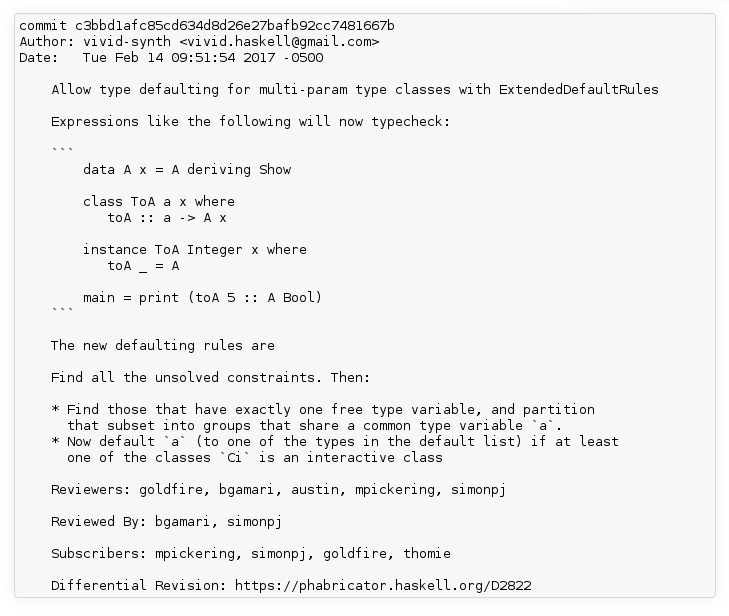FARM 2017

# So what is it?

• Sound synthesis and manipulation in Haskell
• Using the SuperCollider server as a "rendering engine"
• Goal: ergonomic
• Goal: the concrete for houses

# This talk

• Very basics of Vivid
• Design goals
• Implementation successes/failures

# Why SuperCollider?: The Snarky Answer# Why SuperCollider?: The Real Answer

• Amazing community
• Really well-designed
• "Batteries included"
• Optimized real-time batteries (no malloc!)
• Lingua franca
• TidalCycles
• Sonic Pi
• Overtone
• ScalaCollider
• supercollider.js
• SCLang itself
• many, many more

# But it is a spec...

• There are already two (both C++)
• Someone could implement a new spec-compliant real-time server...# Enough talk!: A synth(Thanks, Rohan!)

DAG

# Let's start simple``play \$ 0.1 ~* sinOsc (freq_ 440)``

`sinOsc` represents a binary that's run by the server

``play \$``
• Gets a fresh synth ID from allocator
• Turns the "synth definition" graph to binary
• Construct the OSC message
• Send it over UDP/TCP

# :set -XDataKinds

``````>  :i sinOsc
sinOsc :: Args '["freq"] '["phase"] a => a -> SDBody a Signal``````

All valid:

``sinOsc (freq_ 440)``
``sinOsc (freq_ 440, phase_ 1)``
``sinOsc (phase_ 1, freq_ 440)``

Not valid:

``sinOsc (phase_ 1)``

# Goal: "Morally equivalent"

``(freq_ 440, phase_ 1)``
``HCons (ua 440 :: UA "freq") (HCons (ua 1 :: UA "phase") HNil)``

(Note: HNil isn't too elegant!)

# UGen arguments

``````type family Args (required :: [Symbol])
(optional :: [Symbol])
args
:: Constraint where
Args required optional args =
( Subset required (UAsArgs args)
, Subset (UAsArgs args) (SetUnion required optional)
, FromUA args
)``````

# But that means

So does:

``sinOsc (freq_ 440, phase_ 1)``

...satisfying:

``sinOsc :: Args '["freq"] '["phase"] a => a -> SDBody a Signal``

...mean there's a big file like:

``````freq_ :: ToSig s as => s -> UA "freq" as
phase_ :: ToSig s as => s -> UA "phase" as
-- etc...``````

somewhere?

(`UA` == UGen argument)

Here's why.

# Variants

Any kind of sum type on the args...

``````data Arg =
Freq
| Phase
| -- ...``````

...is out because the set of arguments is an open set

Not only is it possible to write new UGens We want to encourage it!

# Records

One record per UGen...

``````data SinOsc = SinOsc
freq :: x
, phase :: x
}``````

...are out at least until `-XOverloadedRecordFields` is finished

(And also because of `SDBody` problem we'll see later)

Also downside: typing

``{-# LANGUAGE DuplicateRecordFields #-}``

# Record variants

``````data UGen =
SinOsc { freq = x, phase = x }
| Foo { freq = x }``````

Two problems:

• Closed set!
• phase (Foo 0)

# Why the '_'?

``sinOsc (freq_ 440)``

I feel the same way!

Name cluttering:

• "a", "b", "c"
• "freq"
• "x"
• "delay"
• "min"

etc.

"An identifier consists of a letter followed by zero or more letters, digits, underscores, and single quotes." - Haskell 2010 Report

# '_' part 2

Another contender:

``sinOsc (freq'' 440, phase'' 1)``

# Why "freq_" at all?

``foo 440 0.5 1.2 3.5 12``
• Default values
• "Float blindness"

# Boolean blindness

``````  setDoorLocks :: Bool -> Bool -> IO ()
setDoorLocks letPeopleIn letDinosaursOut =``````

"Hmm, what order do they go in again?"

# Float blindness

``someUGen 1 440``

1 = freq 440 = amp

(Also `:i`!)

# Constraints of livecoding

``(0.75::Float) ~* sinOsc (freq_ (440::Float), phase_ (1::Float))``

vs.

``0.75 ~* sinOsc (freq_ 440, phase_ 1)``

(We'll see this again later!)

``length (L.nub "vivid") == 3``# Succinctness Really Matters!# SDBody

Example: "Synth def body"

# Example

These should behave differently

``````do let x = whiteNoise
x ~- x``````
``````do x <- whiteNoise
x ~- x``````

GHC -O2 vs -O0

# Note also

`sinOsc` represents a binary that's run by the server

``sinOsc (freq_ whiteNoise)``
``````do w <- whiteNoise
sinOsc (freq_ w)``````

# SynthDef

• Constants
• Expressions
• Variables?
``````SynthDef(\freq, {
s = SinOsc.ar(freq: freq);
Out.ar(0, s)
})``````

An open set of addressable-by-string identifiers... Hmm...

# Type-level strings again!

``(V::V "amp")``

or

``V @"amp"``

shorter version of

``(Proxy::Proxy "amp")``

# SynthDef

``````x :: SynthDef '["freq"]
x = sd (440 ::I "freq") \$ do
s0 <- sinOsc (freq_ (V::V "freq"), phase_ 0.5)
s1 <- 0.1 ~* s0
out 0 [s1,s1]``````

# 'set'

``````synth ::
(VividAction m, Subset (InnerVars params) args) =>
SynthDef args -> params -> m (Synth args)``````
``````set ::
(VividAction m, Subset (InnerVars params) sdArgs) =>
Synth sdArgs -> params -> m ()``````

See it in action

# Timing

``````class (Monad m , MonadIO m) => VividAction (m :: * -> *) where

callOSC :: OSC -> m ()

newNodeId :: m NodeId

wait :: Real n => n -> m ()

getTime :: m Timestamp

fork :: m () -> m ()

defineSD :: SynthDef a -> m ()

-- And several others...``````

# Demos

``instance VividAction IO``
``instance VividAction Scheduled``
``instance VividAction NRT``

# A law

• All languages have strengths and weaknesses
• A good language makes its strengths easy to write
• In a good language, easy things are fast to write
• A programmer has the most fun working with a language's strengths

Therefore:

• A disproportionate amount of time spent using a good language is spent doing the things it's least fun to do